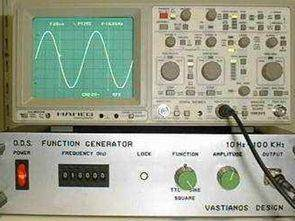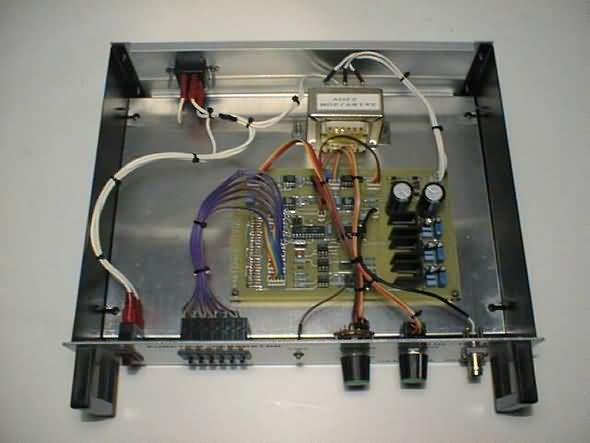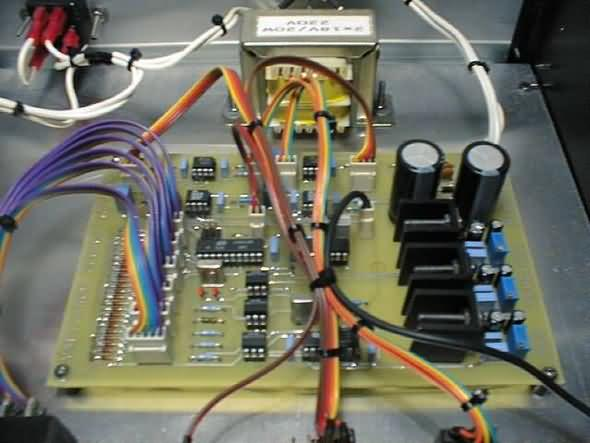# AT90S1200 D.D.S. Function Generator

| Mart 27, 2021 Tarihinde güncellendiThe presented project is a function generator for sinusoidal and square signals production. The output frequency covers the range from 10 Hz to 100 KHz with a step of 2 Hz.

The waveform synthesis is based on the D.D.S. technique (Direct Digital Synthesis) and the output frequency is selected through a microcomputer with an embedded thumb wheel keyboard.

1. Technical specifications

Output Frequency: 10 Hz – 100 KHz (step 2 Hz)
Output Voltage:
Sinus: 0 – 20 Vp-p
Square: 0 – 20 Vp-p
TTL: 5 V
Output Resistance: 1 KOhms

2. Sinusoidal waveform direct digital synthesis

A complete sinusoidal waveform can be synthesized through a D.D.S. system. This system uses a group of 2^21 phases (time slots) and a 16 bit data latch defines how many of them will be contained between two successive synchronization pulses. If the 16 bit data latch contains low numerical values then the output frequency will be low and if the 16 bit data latch contains high numerical values then the output frequency will be high.AT90S1200 D.D.S. Function Generator kaynak: http://www.seattlerobotics.org/encoder/200205/ddsfgen.htm